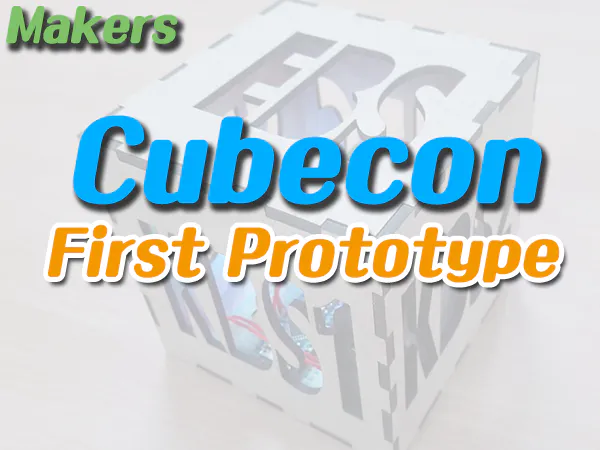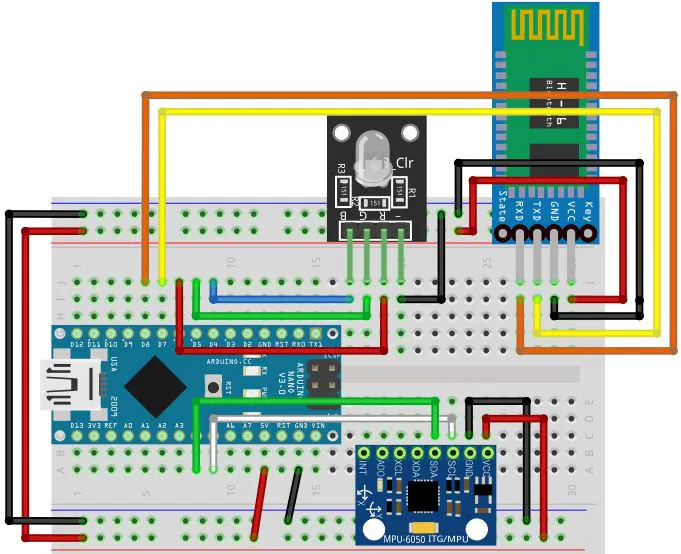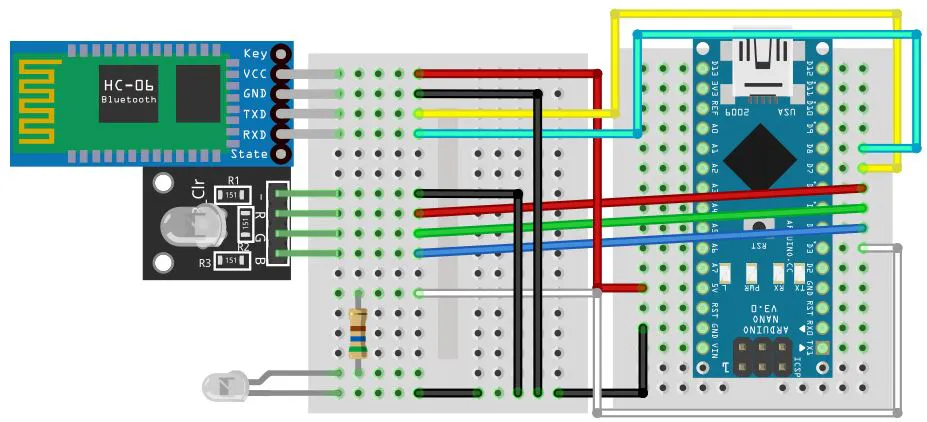Published

# Makers(Cubecon) #4 First Prototype

Bongilcheon High School, Makers club 's team project.

BeginnerWork in progress1 hour886## Things used in this project

### Hardware componentsArduino Nano R3
×2×2
×1
 Arduino MPU-6050
×1
 HC-06 Bluetooth Module
×2
 Arduino RGB LED
×2
 Arduino Infrared transmitter
×1

### Software apps and online servicesArduino IDE

## Schematics

### Cubecon### Signal converter## Code

### Cubecon

Arduino
```#include<Wire.h>
#include<SoftwareSerial.h>

SoftwareSerial btSerial(7, 8);

int16_t GyX, GyY, GyZ;
int red = 6;
int green = 5;
int blue = 4;
String A;
String B;

void setup() {
Wire.begin();
Wire.write(0x6B);
Wire.write(0);
Wire.endTransmission(true);
Serial.begin(9600);
btSerial.begin(9600);
pinMode(red, OUTPUT);
pinMode(green, OUTPUT);
pinMode(blue, OUTPUT);
}

void loop() {
gyro();
check();
led();
}

void gyro() {
Wire.write(0x3B);
Wire.endTransmission(false);
GyX = GyX / 100;
GyY = GyY / 100;
GyZ = GyZ / 100;
Serial.print(F("gyro x,y,z : "));
Serial.print("| GyX = ");
Serial.print(GyX);
Serial.print("| GyY = ");
Serial.print(GyY);
Serial.print("| GyZ = ");
Serial.print(GyZ);
Serial.println();
delay(300);
}

void check() {
// EBS
if (GyX >= -20 && GyX <= -10) {
if (GyY >= -10 && GyY <= 0) {
if (GyZ >= 145 && GyZ <= 155) {
A = "EBS";
}
}
}
// KBS
else if (GyY >= -175 && GyY <= -165) {
if (GyX >= 0 && GyX <= 10) {
if (GyZ >= -20 && GyZ <= -10) {
A = "KBS";
}
}
}
// KBS2
else if (GyX >= 165 && GyX <= 175) {
if (GyY >= -10 && GyY <= 0) {
if (GyZ >= 10 && GyZ <= 20) {
A = "KKBS";
}
}
}
// SBS
else if (GyX >= -155 && GyX <= -145) {
if (GyY >= -5 && GyY <= 5) {
if (GyZ >= -40 && GyZ <= -30) {
A = "SBS";
}
}
}
// MBC
else if (GyX >= 5 && GyX <= 15) {
if (GyY >= 155 && GyY <= 165) {
if (GyZ >= -15 && GyZ <= -5) {
A = "MBC";
}
}
}
else {
A = "X";
}
}

void led() {
if (A == B) {

}
else if (A != B) {
// EBS
if (A == "EBS") {
btSerial.write('A');
digitalWrite(red, HIGH);
digitalWrite(green, HIGH);
digitalWrite(blue, HIGH);
B = "EBS";
}
// KBS
else if (A == "KBS") {
btSerial.write('B');
digitalWrite(red, HIGH);
digitalWrite(green, LOW);
digitalWrite(blue, LOW);
B = "KBS";
}
// KBS2
else if (A == "KKBS") {
btSerial.write('C');
digitalWrite(red, LOW);
digitalWrite(green, HIGH);
digitalWrite(blue, LOW);
B = "KKBS";
}
// SBS
else if (A == "SBS") {
btSerial.write('D');
digitalWrite(red, LOW);
digitalWrite(green, LOW);
digitalWrite(blue, HIGH);
B = "SBS";
}
// MBC
else if (A == "MBC") {
btSerial.write('E');
digitalWrite(red, HIGH);
digitalWrite(green, LOW);
digitalWrite(blue, HIGH);
B = "MBC";
}
}
}
```

### Signal converter

Arduino
The infrared value depends on the remote control.
```#include <SoftwareSerial.h>
#include <boarddefs.h>
#include <IRremote.h>
#include <IRremoteInt.h>
#include <ir_Lego_PF_BitStreamEncoder.h>

SoftwareSerial btSerial(7, 8);
IRsend irsend;

int red = 6;
int green = 5;
int blue = 4;

byte ddata;

void setup() {
Serial.begin(9600);
btSerial.begin(9600);
pinMode(red, OUTPUT);
pinMode(green, OUTPUT);
pinMode(blue, OUTPUT);
}
void loop() {
bluetooth();
IR();
}

void bluetooth() {
byte data;
Serial.println(data);
}

void IR() {

}

else if (adata != ddata) {
// EBS
irsend.sendNEC(0x10EA956, 32);
delay(100);
irsend.sendNEC(0x10EB946, 32);
ddata = 'A';
digitalWrite(red, HIGH);
digitalWrite(green, HIGH);
digitalWrite(blue, HIGH);
}
// KBS
irsend.sendNEC(0x10EF6996, 32);
ddata = 'B';
digitalWrite(red, HIGH);
digitalWrite(green, LOW);
digitalWrite(blue, LOW);
}
// KBS2
irsend.sendNEC(0x10EF7986, 32);
ddata = 'C';
digitalWrite(red, LOW);
digitalWrite(green, HIGH);
digitalWrite(blue, LOW);
}
// SBS
irsend.sendNEC(0x10EF5BA4, 32);
ddata = 'D';
digitalWrite(red, LOW);
digitalWrite(green, LOW);
digitalWrite(blue, HIGH);
}
// MBC
irsend.sendNEC(0x10EFA956, 32);
delay(100);
irsend.sendNEC(0x10EFA956, 32);
ddata = 'E';
digitalWrite(red, HIGH);
digitalWrite(green, LOW);
digitalWrite(blue, HIGH);
}
}
}
```

## Credits

### Yun Jin Yong

6 projects • 8 followers

### Seonggyu Oh

5 projects • 1 follower

### gledel

100 projects • 91 followers
Looking back on my childhood, I was happy when I was making something and I was proud of myself. "Making is instinct!"